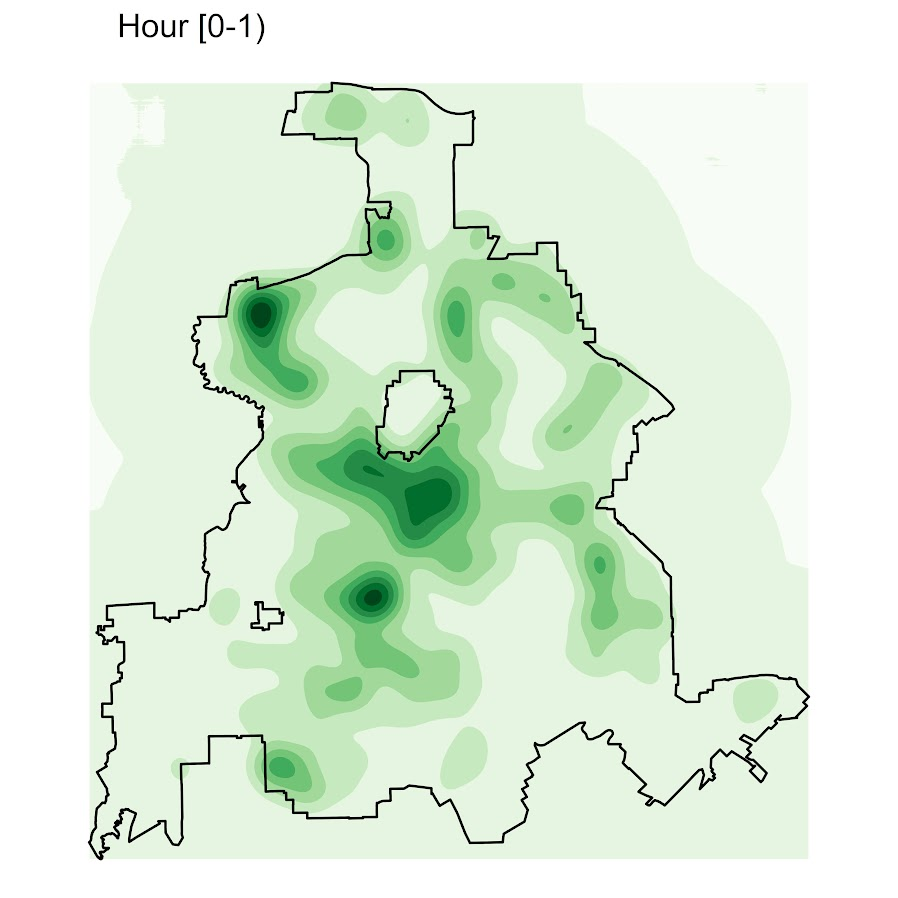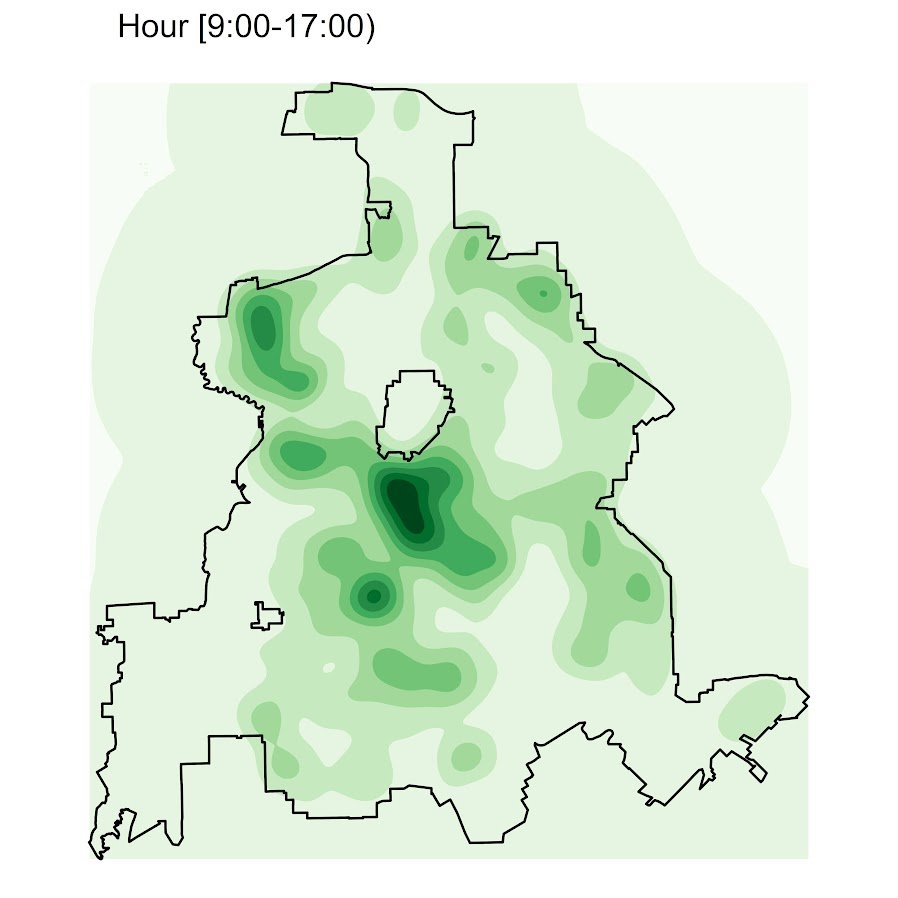# Making aoristic density maps in R

I saw Jerry the other day made/updated an R package to do aoristic analysis. A nice part of this is that it returns the weights breakdown for individual cases, which you can then make maps of. My goto hot spot map for data visualization, kernel density maps, are a bit tough to work with weighted data though in R (tough is maybe not the right word, to use ggplot it takes a bit of work leveraging other packages). So here are some notes on that.

I have provided the data/code here. It is burglaries in Dallas, specifically I filter out just for business burglaries.

# R Code Snippet

First, for my front end I load the libraries I will be using, and change the working directory to where my data is located.

``````############################
library(aoristic) #aoristic analysis
library(rgdal)    #importing spatial data
library(spatstat) #weighted kde
library(raster)   #manipulate raster object
library(ggplot2)  #for contour graphs
library(sf)       #easier to plot sf objects

my_dir <- "D:\\Dropbox\\Dropbox\\Documents\\BLOG\\aoristic_maps_R\\data_analysis"
setwd(my_dir)
############################``````

Next I just have one user defined function, this takes an input polygon (the polygon that defines the borders of Dallas here), and returns a raster grid covering the bounding box. It also have an extra data field, to say whether the grid cell is inside/outside of the boundary. (This is mostly convenient when creating an RTM style dataset to make all the features conform to the same grid cells.)

``````###########################
#Data Manipulation Functions

#B is border, g is size of grid cell on one side
BaseRaster <- function(b,g){
base_raster <- raster(ext = extent(b), res=g)
projection(base_raster) <- crs(b)
mask_raster <- rasterize(b, base_raster, getCover=TRUE) #percentage of cover, 0 is outside
}
###########################``````

The next part I grab the datasets I will be using, a boundary file for Dallas (in which I chopped off the Lochs, so will not be doing an analysis of boat house burglaries today), and then the crime data. R I believe you always have to convert date-times when reading from a CSV (it never smartly infers that a column is date/time). And then I do some other data fiddling – Jerry has a nice function to check and make sure the date/times are all in order, and then I get rid of points outside of Dallas using the sp over function. Finally the dataset is for both residential/commercial, but I just look at the commercial burglaries here.

``````###########################
#Get the datasets

#Geo data
base_Dallas <- BaseRaster(b=boundary,g=200)
base_df <- as.data.frame(base_Dallas,long=TRUE,xy=TRUE)

#Crime Data
#prepping time fields
crime_dat\$Beg <- as.POSIXct(crime_dat\$StartingDateTime, format="%m/%d/%Y %H:%M:%OS")
crime_dat\$End <- as.POSIXct(crime_dat\$EndingDateTime, format="%m/%d/%Y %H:%M:%OS")

#cleaning up data
aor_check <- aoristic.datacheck(crime_dat, 'XCoordinate', 'YCoordinate', 'Beg', 'End')
coordinates(crime_dat) <- crime_dat[,c('XCoordinate', 'YCoordinate')]
crs(crime_dat) <- crs(boundary)
over_check <- over(crime_dat, boundary)
keep_rows <- (aor_check\$aoristic_datacheck == 0) & (!is.na(over_check\$city))
crime_dat_clean <- crime_dat[keep_rows,]

#only look at business burgs to make it go abit faster
busi_burgs <- crime_dat_clean[ crime_dat_clean\$UCROffense == 'BURGLARY-BUSINESS', ]
###########################``````

The next part preps the aoristic weights. First, the `aoristic.df` function is from Jerry’s aoristic package. It returns the weights broken down by 168 hours per day of the week. Here I then just collapse across the weekdays into the same hour, which to do that is simple, just add up the weights.

After that it is some more geographic data munging using the spatstat package to do the heavy lifting for the weighted kernel density estimate, and then stuffing the result back into another data frame. My bandwidth here, 3000 feet, is a bit large but makes nicer looking maps. If you do this smaller you will have a more bumpy and localized hot spots in the kernel density estimate.

``````###########################
#aoristic weights

#This takes like a minute
res_weights <- aoristic.df(busi_burgs@data, 'XCoordinate', 'YCoordinate', 'Beg', 'End')

#Binning into same hourly bins
for (i in 1:24){
cols <- (0:6*24)+i+5
lab <- paste0("Hour",i)
res_weights[,c(lab)] <- rowSums(res_weights[,cols])
}

#Prepping the spatstat junk I need
peval <- rasterToPoints(base_Dallas)[,1:2]
spWin <- as.owin(as.data.frame(peval))
sp_ppp <- as.ppp(res_weights[,c('x_lon','y_lat')],W=spWin) #spp point pattern object

#Creating a dataframe with all of the weighted KDE
Hour_Labs <- paste0("Hour",1:24)

for (h in Hour_Labs){
sp_den <- density.ppp(sp_ppp,weights=res_weights[,c(h)],
sigma=3000,
edge=FALSE,warnings=FALSE)
sp_dat <- as.data.frame(sp_den)
kd_raster <- rasterFromXYZ(sp_dat,res=res(base_Dallas),crs=crs(base_Dallas))
base_df[,c(h)] <- as.data.frame(kd_raster,long=TRUE)\$value
}
###########################``````

If you are following along, you may be wondering why all the hassle? It is partly because I want to use ggplot to make maps, but for its geom_contour it does not except weights, so I need to do the data manipulation myself to supply ggplot the weighted data in the proper format.

First I turn my Dallas boundary into a simple feature `sf` object, then I create my filled contour graph, supplying the regular grid X/Y and the Z values for the first Hour of the day (so between midnight and 1 am).

``````###########################
#now making contour graphs

dallas_sf <- st_as_sf(boundary)

#A plot for one hour of the day
hour1 <- ggplot() +
geom_contour_filled(data=base_df, aes(x, y, z = Hour1), bins=9) +
geom_sf(data=dallas_sf, fill=NA, color='black') +
scale_fill_brewer(palette="Greens") +
ggtitle('       Hour [0-1)') +
theme_void() + theme(legend.position = "none")
hour1

png('Hour1.png', height=5, width=5, units="in", res=1000, type="cairo")
hour1
dev.off()
###########################``````Nice right! I have in the code my attempt to make a super snazzy small multiple plot, but that was not working out so well for me. But you can then go ahead and make up other slices if you want. Here is an example of taking an extended lunchtime time period.

``````###########################
#Plot for the afternoon time period
base_df\$Afternoon <- rowSums(base_df[,paste0("Hour",10:17)])

afternoon <- ggplot() +
geom_contour_filled(data=base_df, aes(x, y, z = Afternoon), bins=9) +
geom_sf(data=dallas_sf, fill=NA, color='black') +
scale_fill_brewer(palette="Greens") +
ggtitle('       Hour [9:00-17:00)') +
theme_void() + theme(legend.position = "none")
afternoon
###########################``````So you can see that the patterns only slightly changed compared to the midnight prior graph.

Note that these plots will have different breaks, but you could set them to be equal by simply specifying a breaks argument in the `geom_contour_filled` call.

I will leave it up so someone who is more adept at R code than me to make a cool animated viz over time from this. But that is a way to mash up the temporal weights in a map.